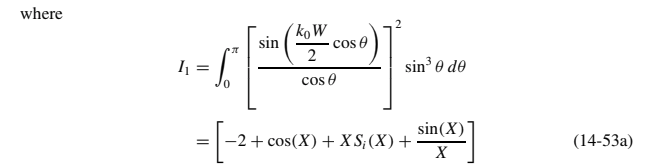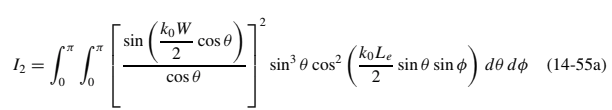# Design A Rectangular Patch Antenna Using Python

22 September 2018

Update 1 : 23/02/2020 Source code of python moved as package patch-antenna-util from normal python file and the post re-organized.

Update 2 : 25/02/2020 Directivity related equations and codes updated in post and also in package patch-antenna-util from normal python file and the post re-organized.

Update 3 : 25/06/2020 Released as python package patch_antenna in pypi and the post organized.

Update 4 : 05/08/2020 Gerber facility added for both python package and live demo.

Contents

### 1. Introduction

Designing a patch antenna has some concepts and mathematical equations. I suggest you to view this Patch antenna concept post, If you are a novice to patch antenna design. In this post, I tried to use python utils and libs for designing a patch antenna.

Normally Python design requires these three important parameter values (As said in suggested post),

• Resonant Frequency (freq)
• Dielectric Constant of the Cavity Material (Er)
• Height of the Dielectric Thickness (h)

### 2. Design Antenna

Especially for rectangular patch antenna the python package : patch-antenna created. Please follow below steps / guidelines for the usage.

###### Installation

Install the required packages scipy and patch_antenna as shown below.

pip install scipy
pip install patch_antenna


Lets us calculate the patch antenna parameters to resonate at 2.4 GHz with the dielectric material having dielectric constant 4.4 and height 1.6 mm.

Define your values in appropriate unit as shown below example code : simple_design.py

import json
import patch_antenna as pa
# resonant frequency in Hz
freq = 2.4 * 10 ** 9
# dielectric constant
er = 4.4
# thickness of the cavity in meter
h = 1.6 * 10 ** -3
result = pa.design(freq, er, h)
# pretty printing
print(json.dumps(result, indent=4))

##### Output

The output of the code is just pretty printed using package json. All calculated results are in their base unit such as,

• frequency in Hz
• impedance in ohm
• All design lengths are in meter.

{ “frequency”: 2400000000.0, “patch_width”: 0.0380099749575278, “patch_length”: 0.0294215930843705, “feeder_width”: 0.015203989983011122, “feeder_length”: 0.015449608708025277, “inset_gap_width”: 0.007601994991505561, “inset_length”: 0.010914409094654586, “ground_length”: 0.05447120179239577, “ground_width”: 0.06281396494053892, “input_edge_impedance”: 321.50075290241097 }

##### Gerber facility

The design can be stored as gerber file, which is commonly required for cnc fabrication.

• Normal feed
pa.write_gerber(freq, er, h, 'patch_design_normal_2.4GHz_4.4_er_1.6_h.gbr', 'normal')

• Inset feed
pa.write_gerber(freq, er, h, 'patch_design_inset_2.4GHz_4.4_er_1.6_h.gbr', 'inset')


### 3. Description

##### Patch Antenna Feed Types

There are multiple types of feeding to patch antenna. This design output is used for these two types shown below,

Patch antenna feeding types##### Optimal Inset Feed position (inset_length)

Optimal inset feed length calculated to reduce the input impedance of feeding point to the patch. This optimal point can be calculated from the standard impedance 50 Ohm and length of the designed patch antenna.

Image of Voltage (V), current (I), impedance (Z) curve along the length of patchNote:

Basic design steps and related details are completed here. Hope you got your antenna design. Following contents use another development github repository Patch-antenna-util. If you wish to know more about this, proceed the following.

### 4. Other Parameters

Other parameters such as directivity, EHPlane and Surface plot are organized in the another repository Patch antenna util. Follow below steps if the above discussed details required otherwise skip this.

##### Initialize source code

Clone the repository Patch antenna util and update your python path by running the command,

git clone https://github.com/Bhanuchander210/patch-antenna-util.git cd patch-antenna-util export PYTHONPATH=\$PYTHONPATH:/path/to/patch_util

##### Python script

Then modify design_patch.py or write your own python code as shown below.

Import the patch_util package and initialize the your parameters to its variables such as frequency, dielectric constant and thickness of the cavity.

from patch_util.patch import get_directivity, patch_eh_plane_plot, surface_plot, get_i1
freq = 2.4e9
er = 4.4
h = 1.6* 10 ** -3

##### Directivity

Directivity is the metric which explains about the Radiation Pattern of the antenna. Directivity is the ratio of the maximum radiation intensity of a particular direction to the normalized radiation intensity of all direction.Image Source : Research Gate

According to the reference Antenna Theory: Analysis and Design by Constantine A. Balanis : Chapter 14.2.3, There are two kind of equations (14-53a, 14-55a) which is used to calculate the value I for different cases single slot and two slot.So that we can get two different directivity values as d1 and d2 calculated from i1, i2 respectively. The script to calculate i1 value has been implemented as method get_i1() when the other one i2 skipped because of complexity.

patch_width = result['patch_width']
i1 = get_i1(patch_width, freq)
print("The value for equation (14-53a) : ", i1)


The value for equation (14-53a) : 1.1479757280698542

Then the second value i2 assumed here which is need to be calculated manually from the equation 14-55a. The directivity calculation methods are,

d1 = get_directivity(patch_width, freq, patch_length)
print('Directivity : ', d1, ' dB')

# Let's assume the value i2
i2 = 2
d2 = get_directivity_two_slot(W, freq, i2)
print("Directivity (two-slot) : ", d2, ' dB')


Directivity : 5.9869953652616035 dB Directivity (two-slot) : 7.59055858259433 dB

##### Fields of Electric and Magnetic Plane - Plot

fields = patch_eh_plane_plot(freq, W, L, h, Er)##### Surface Plot - 3D

surface_plot(fields)